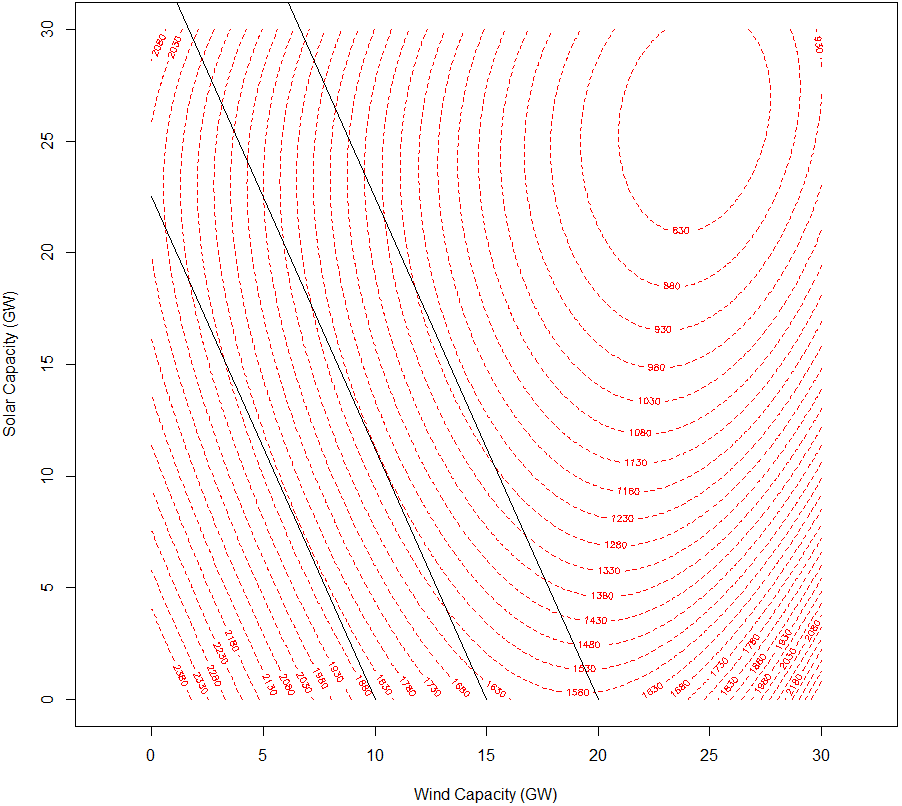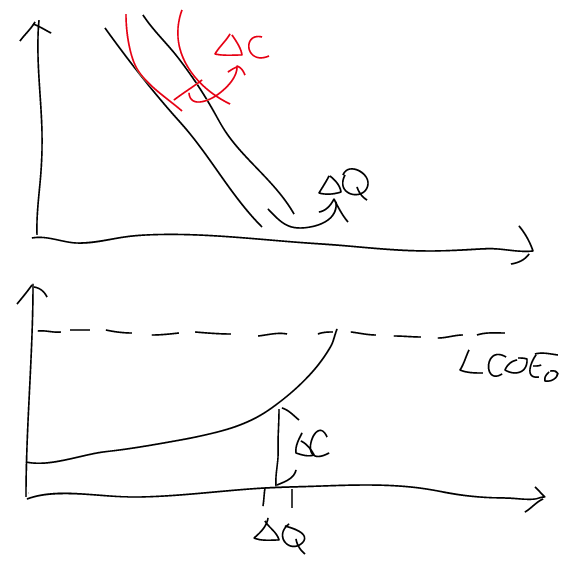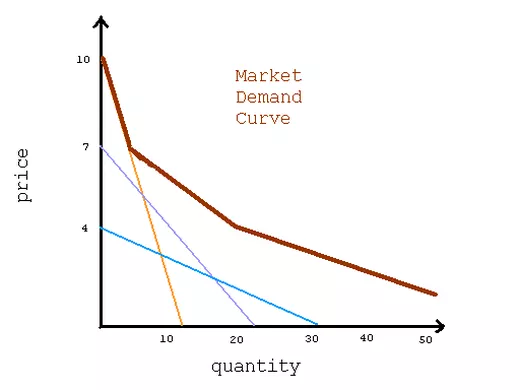# All the Efficiencies You Need to Know about Economy

The other day when our social economy teacher is teaching equimarginal principle, and stated that this principle would automatically lead to efficiency.

This came into my alarm radar, because a year ago in an environmental economy class the professor explicitly reminded us again and again equimarginal principle only guaranteed cost effectiveness but not economy efficiency. To show how highly she regarded this concept, she even had it on the exam!

After I did some research and review on economics 101, I realized that both claims were correct in the sense that the efficiency they were talking about were different concepts. So I think it is time I write an article about this.

# Four Types of Efficiency in Economics

There are four types of efficiency in economics: the productive efficiency, the cost efficiency, the consumer efficiency, and the economy efficiency. Their overall relationship are depicted in the following diagram.

So we can see that cost efficiency is a subset of productive efficiency, and economic efficiency is the union of consumer efficiency and cost efficiency. These efficiency concepts are all Pareto efficient with regard to the concerned utility functions or preference.

# Productive Efficiency

The first and loosest efficiency is the productive efficiency. As shown in the wiki page, under constrained technology and resources, there will be a maximum level of achievable production, forming a production-possibility frontier. Productive efficiency is reached when the production is on the frontier.

Let us take the following example. Suppose there are two options for a policy maker during the early stage of energy transition: to build solar capacity or to build wind capacity, under the constraint that the total budget for VRE is capped at C=100.

Assume that wind and solar have the followingly total cost profile, respectively:

Plotting the corresponding wind and solar capacity at each possible budget combinations, we would get the production-possible frontier:

It is important to know that PPF can be a 2-D mesh or even in a higher dimension, and the principle is still the same. For example, we get add also other investment options such as demand response, storage, flexibility improvements, and dispatchable conventional sources into the domain space.

# Cost Efficiency

Productive efficiency only guarantees that the producer uses up the resource as much as she is allowed to. However, for a certain type of objective function T (i.e., the profit of a producer), there might exist an optimum combination on the PPF such that the objective function is maximized. Also, such objective function can help us define a marginal opportunity cost function.

Cost efficiency occurs when the producer chooses to produce at the optimum point. Cost efficiency is also sometimes called cost effectiveness, and it follows the equi-marginal principle, since at optimum point we have

Note that for more than two types of production, when one type of product is increased, there will be an infinite number of possible linear combinations of infinitesimal changes of the remaining variables. According to the definition of marginal opportunity cost, we should find the linear combination such that the magnitude of the infinitesimal change of the objective function is maximized, that is to

In the above example, suppose we want to minimize the total system cost of different VRE deployment combinations.

The integration cost and the system LCoE of VRE is illustrated in the graph below. Note that this type of calculation does not count the optimization of flexibility improvements, DR and other options of integration into the integration costs of VRE.

Suppose we have the system LCoE of VRE as the following:

The total VRE electricity generation is

And the total system cost, given total electricity demand is 1000, is

We can then plotted the corresponding contour along with the PPF, and clearly sees that TC=1400 is the optimum under the conditions we have made. The optimum capacity for both solar and wind are about 13 GW.

It is also worth noting that the cost efficiency problem for different budget constraints can lead to an optimum dynamic path as more money is invested. For instance, consider our example when c grows from 100 to 110:

The optimum total system cost is then 1330, and there should be about 1GW of addition capacity for both wind and solar to achieve such new optimum.

Note that even applying this optimum path, one cannot guarantee that her profit is maximized. For instance, the average electricity price on the wholesale market will affect the profits also. Cost efficiency, or cost effectiveness, just guarantees that the unit of production with the least marginal opportunity cost is always produced first, so the total cost will be minimized under certain constraints.

The supply curve can also be derived from this analysis. Plotting the iso-electricity generation lines will give us the optimum combination of VRE sources for any desired electricity generation VRE, with an optimum total system cost. If we want to increase the electricity generation of VRE for 1 unit, then a new optimum total system cost would be derived, and their difference is the marginal production cost of one unit of VRE electricity generation on the supply curve.The lines indicate constant electricity generation of 450, 675, and 900The relationship of the total cost contour plot and the supply curve. The total cost for any given electricity demand is minimized when VRE electricity is produced until its marginal cost equals that of the conventional power system.

# Consumer Efficiency

An parallel analogy of cost efficiency follows in the consumer’s case: consumer efficiency happens when every good is distributed to the individual who has the greatest marginal utility for the good.

If that is the case, than the total utility gain of all the consumers is maximized for any given amount of distributed goods.

The marginal utility is usually the willingness to pay on the demand curve, and we can easily verify that the aggregate demand curve is a result of consumer efficiency.The aggregate demand curve is a result of consumer efficiency, because the curve shows the highest willingness to pay of all the consumers in the market at any given quantity.

# Economic Efficiency

Suppose a quantity of products are produced in the minimum cost (cost efficiency), and each unit of product is distributed to the consumers who has the highest marginal utility gain for that unit (consumer efficiency). That means that for any given quantity of produced goods, we have the maximum total social welfare gain.

After comparing this results at different level of quantity, we can go one step further and find the global maximum of total social welfare. When this global maximum is reached, we called the system economic efficient.

As taught in every elementary economic class, economic efficient happens when the highest possible marginal utility gain of a product equals to the lowest possible marginal cost of it. On a graph this is the intersection of the supply curve and demand curve.

# Analogy to Statistic Mechanics

We have finished introducing the main concepts of this article, but for those of you who know also a little bit about statistic mechanics, there is a parallel between types of efficiency in economy and types of ensembles in stat mech.

In statistic mechanics, the objective function is to maximize the entropy of a system. When there given the total energy and particle number in a system, we have a microcanonical ensemble; when the system is allowed to exchange energy with the environment we have the ordinary canonical ensemble; when the particles are also allowed to be exchanged we have the grand canonical ensemble.

This means that the optimum problem of the microcanonical ensemble is to maximize entropy, OCE is to maximize entropy with respect to energy change, and GCE is to maximize entropy with respect to both energy and particle change.

--

--